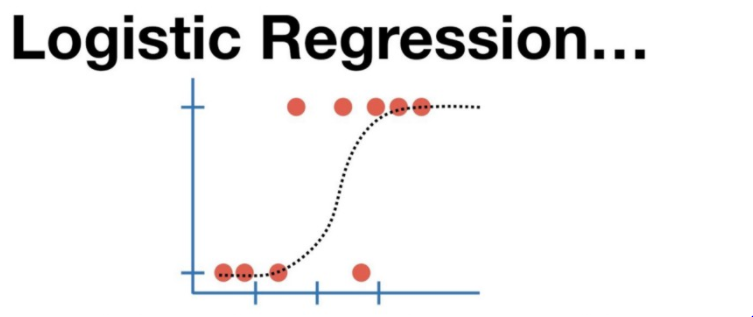# Logistic Regression Math & Geometrical Intuition with ExampleLogistic Regression Math & Geometrical Intuition with Example. Logistic Regression is a Classifier which is used to solve the classification problems. As it’s technically dependent on the Linear Regression & Logit function is a method for a classification problem.

Logistic Regression is the appropriate regression analysis to conduct when the dependent variable is dichotomous (binary). Like all regression analyses, logistic regression is a predictive analysis. Logistic regression is used to describe data and to explain the relationship between one dependent binary variable and one or more nominal, ordinal, interval, or ratio-level independent variables.

Logistic Regression is a Classifier which is used to solve the classification problems. As it’s technically dependent on the Linear Regression & Logit function is a method for a classification problem.

Why Can’t we use Linear Regression for Classification?

If everything is fine we can use linear regression, why logistic regression is needed.

a. Outlier: If the data set has an outlier, linear regression will not perform better.

b. High-end classification: Can be classified as >1 or <0 which is an issue

## Logistic Regression in Classification model using Python: Machine Learning

Learning how to build a basic logistic regression model in machine learning using python . Logistic regression is a commonly used model in various industries such as banking, healthcare because when compared to other classification models, the logistic regression model is easily interpreted.

## Linear Regression Model for Machine Learning

An overview of the oldest supervised machine-learning algorithm, its type & shortcomings.

## Logistic Regression: Discover the Powerful Classification Technique

Logistic Regression: Discover the Powerful Classification Technique. Get to know one of the most widely used classification techniques.

## Most popular Data Science and Machine Learning courses — July 2020

Most popular Data Science and Machine Learning courses — August 2020. This list was last updated in August 2020 — and will be updated regularly so as to keep it relevant

## PySpark in Machine Learning | Data Science | Machine Learning | Python

PySpark in Machine Learning | Data Science | Machine Learning | Python. PySpark is the API of Python to support the framework of Apache Spark. Apache Spark is the component of Hadoop Ecosystem, which is now getting very popular with the big data frameworks.